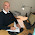## torsdag 8 oktober 2020

### What is a 2020 Nobel Physics Prize Singularity?

The motivation 2020 Nobel Physics Prize awarded Roger Penrose reads:

• In January 1965, ten years after Einstein’s death, Roger Penrose proved that black holes really can form and described them in detail; at their heart, black holes hide a singularity in which all the known laws of nature cease. His ground-breaking article is still regarded as the most important contribution to the general theory of relativity since Einstein.
• Penrose proceeded to prove that once a trapped surface had formed, it is impossible, within the theory of general relativity and with a positive energy density, to prevent the collapse towards a singularity (Penrose 1965).
A singularity is thus described as something in which all the known laws of nature cease.

Let us try to understand what the meaning of such statement can be, and let first recall that mathematics is filled with singularities, and that there are ways to handle such things. As a typical example, take a look att the function $f(x)$ defined by
• $f(x) = 0$ for $x < 0$
• $f(x) = 1$ for $x > 0$,
with the value for x = 0 left unspecified. This is a function which "jumps" from 0 to 1 at the point $x = 0$ with a derivative which appears to be infinite. Nothing very strange, although you may recall that
•  Natura non facit saltus.   (Nature does not jump)  (Axiom of Leibniz)
meaning that the physical jump takes place over a certain distance with a large derivative.

In any case, you may say that the function $f(x)$ "ceases to exist" for x = 0, since no value is specified. But there is something, which is well defined, and that is the jump ( = 1). So there is a form of singularity, where the function f(x) ceases to exist/is not defined, but still the jump of f(x) exists and is well defined.

There is a well developed mathematical theory to deal with singularities and jumps, which is called distribution theory. In this theory the properties the function f(x) would be expressed through integrals
• $\int f(x)\phi (x) dx$
where $\phi (x)$ is any smooth test function. The jump of f(x) would then come out as
• $-\int f(x)\phi^\prime (x)\, dx = \phi (0)$  where $\phi^\prime = \frac{d\phi}{dx}$ is the derivative of $\phi$,
which can be phrased as: The derivative $f^\prime (0)$ is a delta function at 0, which gives a precise meaning to a derivative which appears to be infinite in a standard pointwise sense.

A (unit) point mass is an elementary concept in mechanics, like a very small ball of very high density with total mass = 1, which can be represented by a delta function.  So a point mass is a singularity without any mystery whatsoever, and it would be futile to ask for any internal physics of a such a thing, or to say that inside a point mass laws of physics cease.

In mathematics singularities thus can have well defined properties (expressing laws of physics), and so a singularity may not be all that mysterious and without any physics!  Why is then the singularity discovered by Penrose worth a Nobel Prize?

Maybe then after all, physics does not cease to exist, because physics cannot cease to exist: The show must go on. (Leibniz).

#### 1 kommentar:

1.Very well said, professor!